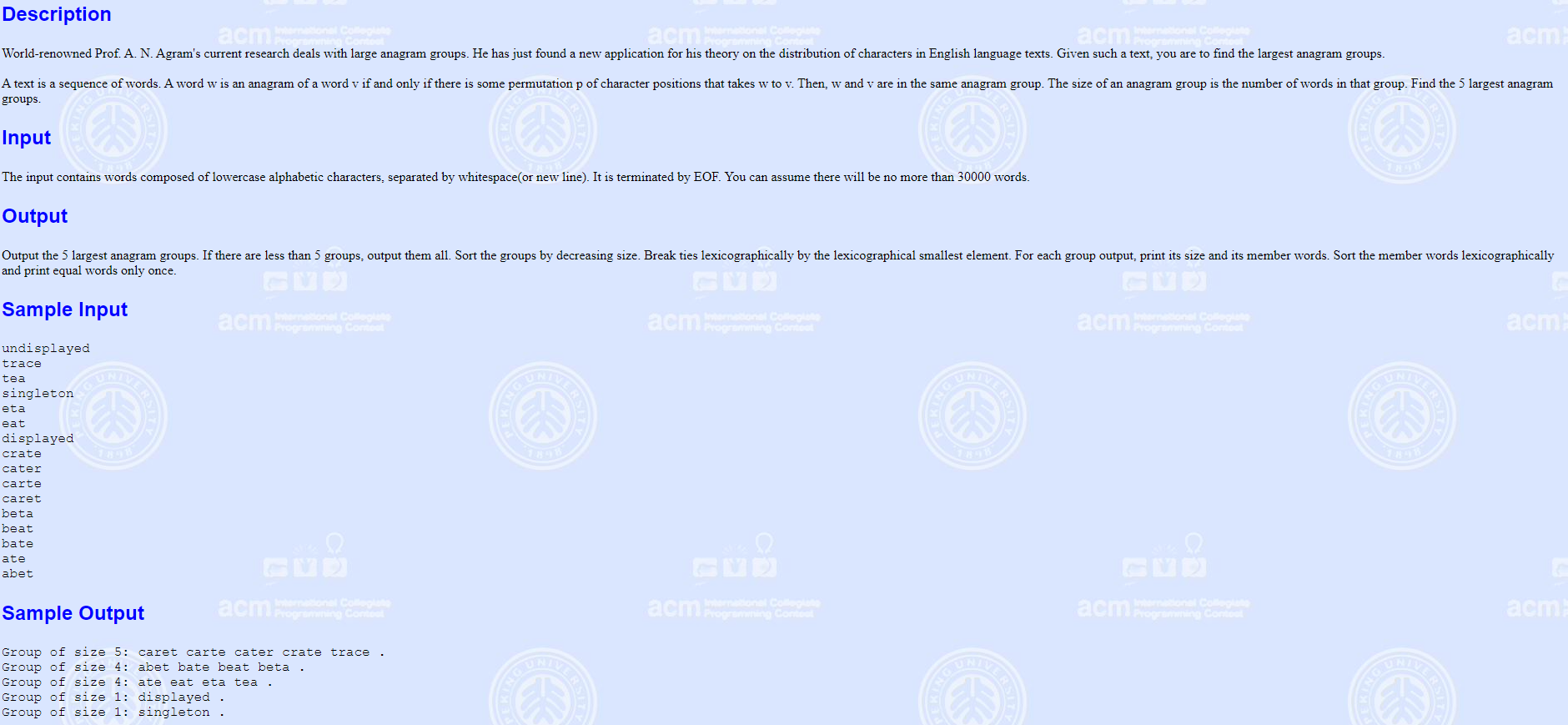# POJ 2408 Anagram Groups（Trie+思维）1. 题目可以用 map+set 过，但是常数较大，我们采用字典树，将每一个单词在树上的标记作为桶
2. 将不同的字符串转化为字典序最小的，可以采用快排，这里采用基数排序
3. 可能是 set 里面有 const 修饰的形参，所以 cmp 函数必须采用 const 的形参
const int N=1e6+5;

int n,m;
int i,j,k;
char s[N];
int t[N],tot=0;
struct Node
{
set<string> all;
int sz;
}vis[N];
int digit,id[N],ans=0;

int idx(char ch){ return ch-'a'; }

void insert(char *s)
{
string str="";
int len=strlen(s);
for(int i=0;i<s[i];i++) digit[s[i]-'a']++; //基数排序
for(int i=0;i<26;i++) while(digit[i]) str+=i+'a',--digit[i];
int rt=0;
for(int i=0;i<str[i];i++) {
int x=idx(str[i]);
if(!t[rt][x]) t[rt][x]=++tot;
rt=t[rt][x];
}
if(vis[id[rt]].sz==0) id[rt]=++ans;
vis[id[rt]].sz++; vis[id[rt]].all.insert((string)s);
}

bool cmp(const Node &a,const Node &b)
{
return a.sz==b.sz?(*(a.all.begin()))<(*(b.all.begin())):a.sz>b.sz;
}

int main()
{
//IOS;
while(scanf("%s",s)!=EOF) insert(s);
set<string>::iterator it;
sort(vis+1,vis+1+ans,cmp);
//for(it=vis.all.begin();it!=vis.all.end();it++) dbg(*it);
for(int i=1;i<=5;i++){
printf("Group of size %d: ",vis[i].sz);
for(it=vis[i].all.begin();it!=vis[i].all.end();it++) printf("%s ",(*it).c_str());
puts(".");
}
PAUSE;
return 0;
}

08-09908
06-1319709-1173
08-075768
08-15680
02-17269
07-10120
10-062035
01-14911
02-2990
04-19